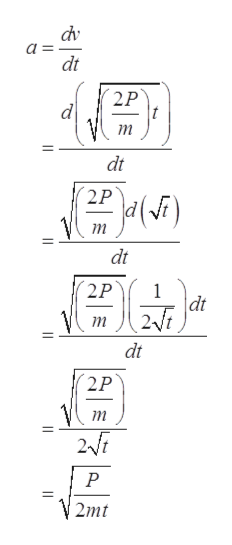# When a 1984 Alfa Romeo Spider sports car accelerates at the maximum possible rate, its motion during the first 20 s is extremely well modeled by the simple equation: v2x =(2P/m)*twhere P=3.6×104 W is the car's power output, m = 1200kg is its mass, and vx is in m/s. That is, the square of the car's velocity increases linearly with timeFind a symbolic expression, in terms of P, m, and t, for the car's acceleration at time t.

Question

When a 1984 Alfa Romeo Spider sports car accelerates at the maximum possible rate, its motion during the first 20 s is extremely well modeled by the simple equation: v2x =(2P/m)*t
where P=3.6×104 W is the car's power output, m = 1200kg is its mass, and vx is in m/s. That is, the square of the car's velocity increases linearly with time

Find a symbolic expression, in terms of P, m, and t, for the car's acceleration at time t.

check_circleExpert Solution
Step 1

Given,

Step 2

The velocity of the car,

Step 3

Now, acceleratio...help_outlineImage Transcriptionclosedv dt 2P d т dt 2P т dt 2P dt 2/i т dt 2P m 2fi Р 2mt fullscreen

### Want to see the full answer?

See Solution

#### Want to see this answer and more?

Solutions are written by subject experts who are available 24/7. Questions are typically answered within 1 hour*

See Solution
*Response times may vary by subject and question
Tagged in

### Kinematics